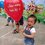# Need some helps!

Find the value of $x$:
$x^2$ + ($\frac {x} {x-1}$)$^2$ = $\frac {5} {4}$Note by Mục Xiên
6 years, 6 months ago

This discussion board is a place to discuss our Daily Challenges and the math and science related to those challenges. Explanations are more than just a solution — they should explain the steps and thinking strategies that you used to obtain the solution. Comments should further the discussion of math and science.

When posting on Brilliant:

• Use the emojis to react to an explanation, whether you're congratulating a job well done , or just really confused .
• Ask specific questions about the challenge or the steps in somebody's explanation. Well-posed questions can add a lot to the discussion, but posting "I don't understand!" doesn't help anyone.
• Try to contribute something new to the discussion, whether it is an extension, generalization or other idea related to the challenge.

MarkdownAppears as
*italics* or _italics_ italics
**bold** or __bold__ bold
- bulleted- list
• bulleted
• list
1. numbered2. list
1. numbered
2. list
Note: you must add a full line of space before and after lists for them to show up correctly
paragraph 1paragraph 2

paragraph 1

paragraph 2

[example link](https://brilliant.org)example link
> This is a quote
This is a quote
    # I indented these lines
# 4 spaces, and now they show
# up as a code block.

print "hello world"
# I indented these lines
# 4 spaces, and now they show
# up as a code block.

print "hello world"
MathAppears as
Remember to wrap math in $$ ... $$ or $ ... $ to ensure proper formatting.
2 \times 3 $2 \times 3$
2^{34} $2^{34}$
a_{i-1} $a_{i-1}$
\frac{2}{3} $\frac{2}{3}$
\sqrt{2} $\sqrt{2}$
\sum_{i=1}^3 $\sum_{i=1}^3$
\sin \theta $\sin \theta$
\boxed{123} $\boxed{123}$

Sort by:

I expanded it, and by hit and trial method, -1 was the solution. Then I divided the expanded polynomial by (x+1). The resultant cubic can be easily factorised.

- 6 years, 6 months ago

$x = y + 1$

$( y + 1)^2 + (\dfrac{y + 1}{y})^2 = \dfrac{5}{4}$

$y^2 + 2y + 1 + 1 + \dfrac{1}{y^2} + \dfrac{2}{y} = \dfrac{5}{4}$

$y^2 + \dfrac{1}{y^2} + 2y + \dfrac{2}{y} + 2 = \dfrac{5}{4}$

$a = y + \dfrac{1}{y}$

$a^2 - 2 + 2a + 2 = \dfrac{5}{4}$

$(a + 1)^2 = (\pm \dfrac{3}{2})^2$

$a = - \dfrac{5}{2} , \dfrac{1}{2}$

$y + \dfrac{1}{y} = - \dfrac{5}{2} , y + \dfrac{1}{y} = \dfrac{1}{2}$

$y = \dfrac{ -\dfrac{5}{2} \pm \sqrt{\dfrac{9}{4}}}{2} , y = \dfrac{ \dfrac{1}{2} \pm i\sqrt{\dfrac{15}{4}}}{2}$

$x - 1 = \dfrac{ -\dfrac{5}{2} \pm \sqrt{\dfrac{9}{4}}}{2} , x - 1 = \dfrac{ \dfrac{1}{2} \pm i\sqrt{\dfrac{15}{4}}}{2}$

$(x - 1= \dfrac{-1}{2} , -4) ~or~ x - 1 = \dfrac{ \dfrac{1}{2} \pm i\sqrt{\dfrac{15}{4}}}{2}$

$x = \dfrac{1}{2} , -3 , \dfrac{3 \pm i\sqrt{15}}{4}$

- 6 years, 6 months ago

x= -1 or 1/2

- 6 years, 6 months ago

Can I know how to solve this, please?

- 6 years, 6 months ago

What have you tried?

Do you know how to clear denominators and factorize?

Staff - 6 years, 6 months ago

I've already cleared denominators and got
$4x^2$ - $8x^3$ + $3x^2$ + $10x$ - $5$ = $0$
What to do next?

- 6 years, 6 months ago

well, we want to start to factorize the polynomial. You can use the rational root theorem
to guess at possible roots, and after which use the remainder factor theorem to help factorize. Have you seen these theorems before?

Staff - 6 years, 6 months ago

Well, I think I haven't seen these theorems before. Because I'm a middle school students. BTW, I'll ask my teacher for this problem's explaination, thanks for your helps!

- 6 years, 6 months ago